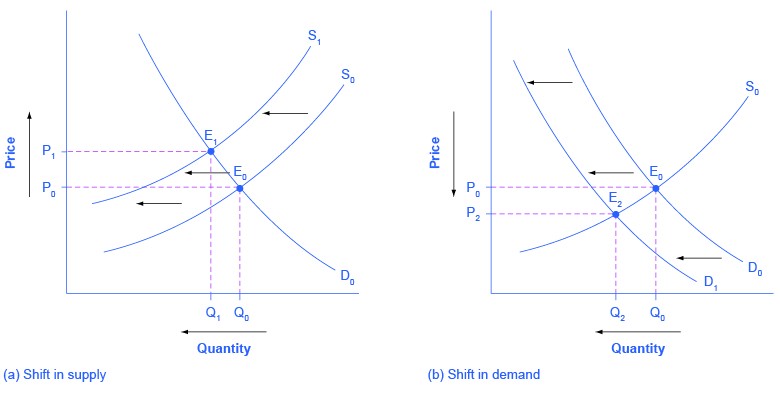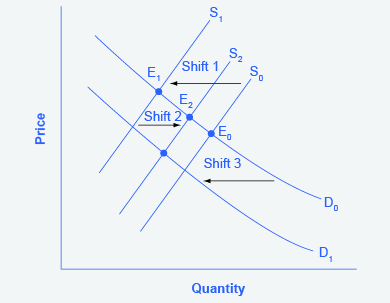# Changes in Equilibrium Price and Quantity: The Four-Step Process

## A Combined Example

The U.S. Postal Service is facing difficult challenges. Compensation for postal workers tends to increase most years due to cost-of-living increases. At the same time, increasingly more people are using email, text, and other digital message forms such as Facebook and Twitter to communicate with friends and others. What does this suggest about the continued viability of the Postal Service? Figure and the text below illustrate this using the four-step analysis to answer this question.Higher Compensation for Postal Workers: A Four-Step Analysis (a) Higher labor compensation causes a leftward shift in the supply curve, a decrease in the equilibrium quantity, and an increase in the equilibrium price. (b) A change in tastes away from Postal Services causes a leftward shift in the demand curve, a decrease in the equilibrium quantity, and a decrease in the equilibrium price.

Since this problem involves two disturbances, we need two four-step analyses, the first to analyze the effects of higher compensation for postal workers, the second to analyze the effects of many people switching from “snail mail” to email and other digital messages.

Figure (a) shows the shift in supply discussed in the following steps.

Step 1. Draw a demand and supply model to illustrate what the market for the U.S. Postal Service looked like before this scenario starts. The demand curve D0 and the supply curve S0 show the original relationships.

Step 2. Did the described change affect supply or demand? Labor compensation is a cost of production. A change in production costs caused a change in supply for the Postal Service.

Step 3. Was the effect on supply positive or negative? Higher labor compensation leads to a lower quantity supplied of postal services at every given price, causing the supply curve for postal services to shift to the left, from S0 to S1.

Step 4. Compare the new equilibrium price and quantity to the original equilibrium price. The new equilibrium (E1) occurs at a lower quantity and a higher price than the original equilibrium (E0).

Figure (b) shows the shift in demand in the following steps.

Step 1. Draw a demand and supply model to illustrate what the market for U.S. Postal Services looked like before this scenario starts. The demand curve D0 and the supply curve S0 show the original relationships. Note that this diagram is independent from the diagram in panel (a).

Step 2. Did the change described affect supply or demand? A change in tastes away from snail mail toward digital messages will cause a change in demand for the Postal Service.

Step 3. Was the effect on demand positive or negative? A change in tastes away from snailmail toward digital messages causes lower quantity demanded of postal services at every given price, causing the demand curve for postal services to shift to the left, from D0 to D1.

Step 4. Compare the new equilibrium price and quantity to the original equilibrium price. The new equilibrium (E2) occurs at a lower quantity and a lower price than the original equilibrium (E0).

The final step in a scenario where both supply and demand shift is to combine the two individual analyses to determine what happens to the equilibrium quantity and price. Graphically, we superimpose the previous two diagrams one on top of the other, as in Figure.Combined Effect of Decreased Demand and Decreased Supply Supply and demand shifts cause changes in equilibrium price and quantity.

Following are the results:

Effect on Quantity: The effect of higher labor compensation on Postal Services because it raises the cost of production is to decrease the equilibrium quantity. The effect of a change in tastes away from snail mail is to decrease the equilibrium quantity. Since both shifts are to the left, the overall impact is a decrease in the equilibrium quantity of Postal Services (Q3). This is easy to see graphically, since Q3 is to the left of Q0.

Effect on Price: The overall effect on price is more complicated. The effect of higher labor compensation on Postal Services, because it raises the cost of production, is to increase the equilibrium price. The effect of a change in tastes away from snail mail is to decrease the equilibrium price. Since the two effects are in opposite directions, unless we know the magnitudes of the two effects, the overall effect is unclear. This is not unusual. When both curves shift, typically we can determine the overall effect on price or on quantity, but not on both. In this case, we determined the overall effect on the equilibrium quantity, but not on the equilibrium price. In other cases, it might be the opposite.

The next Clear It Up feature focuses on the difference between shifts of supply or demand and movements along a curve.

## What is the difference between shifts of demand or supply versus movements along a demand or supply curve?

One common mistake in applying the demand and supply framework is to confuse the shift of a demand or a supply curve with movement along a demand or supply curve. As an example, consider a problem that asks whether a drought will increase or decrease the equilibrium quantity and equilibrium price of wheat. Lee, a student in an introductory economics class, might reason:

“Well, it is clear that a drought reduces supply, so I will shift back the supply curve, as in the shift from the original supply curve S0 to S1 on the diagram (Shift 1). The equilibrium moves from E0 to E1, the equilibrium quantity is lower and the equilibrium price is higher. Then, a higher price makes farmers more likely to supply the good, so the supply curve shifts right, as shows the shift from S1 to S2, shows on the diagram (Shift 2), so that the equilibrium now moves from E1 to E2. The higher price, however, also reduces demand and so causes demand to shift back, like the shift from the original demand curve, D0 to D1 on the diagram (labeled Shift 3), and the equilibrium moves from E2 to E3.”Shifts of Demand or Supply versus Movements along a Demand or Supply Curve A shift in one curve never causes a shift in the other curve. Rather, a shift in one curve causes a movement along the second curve.